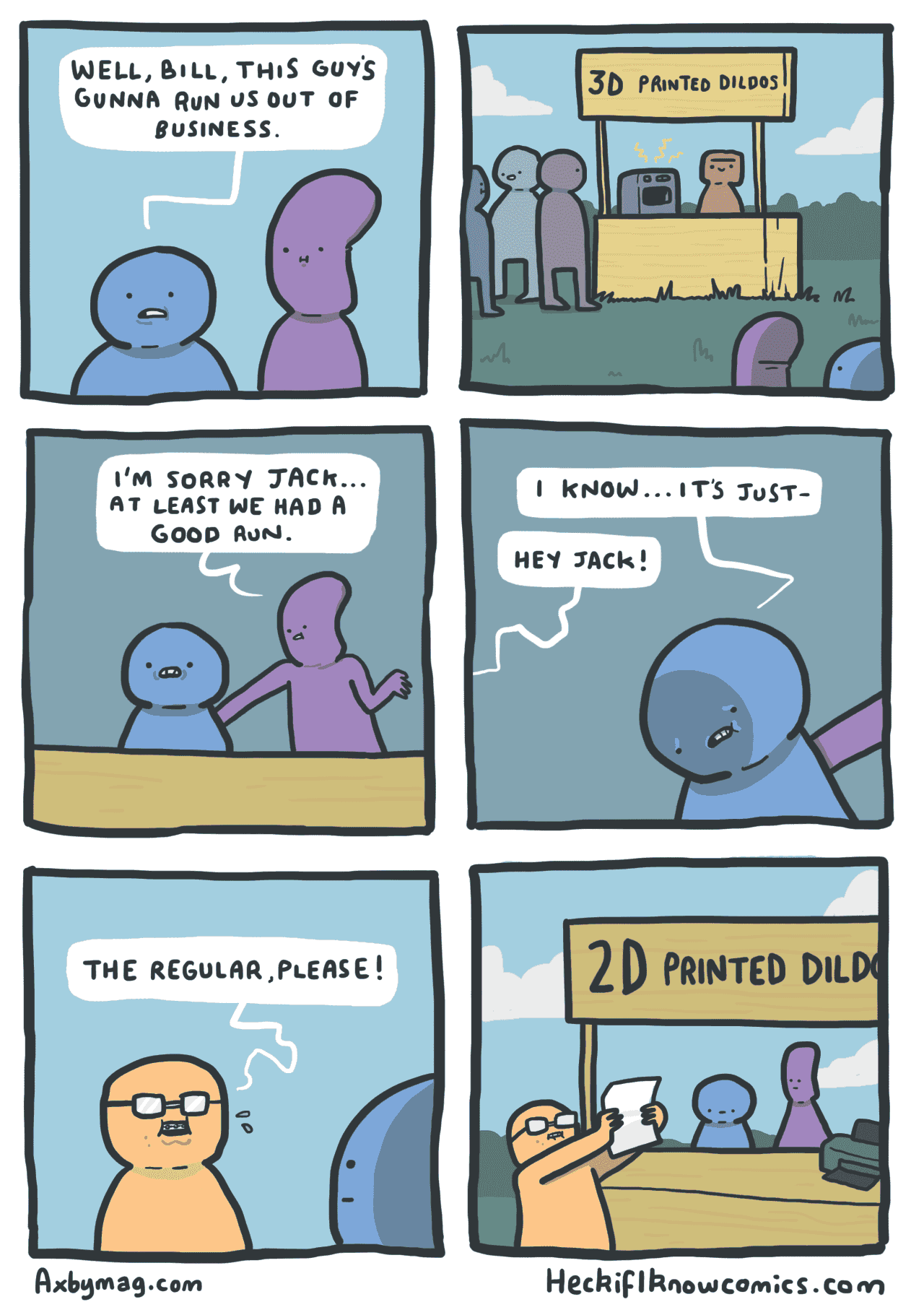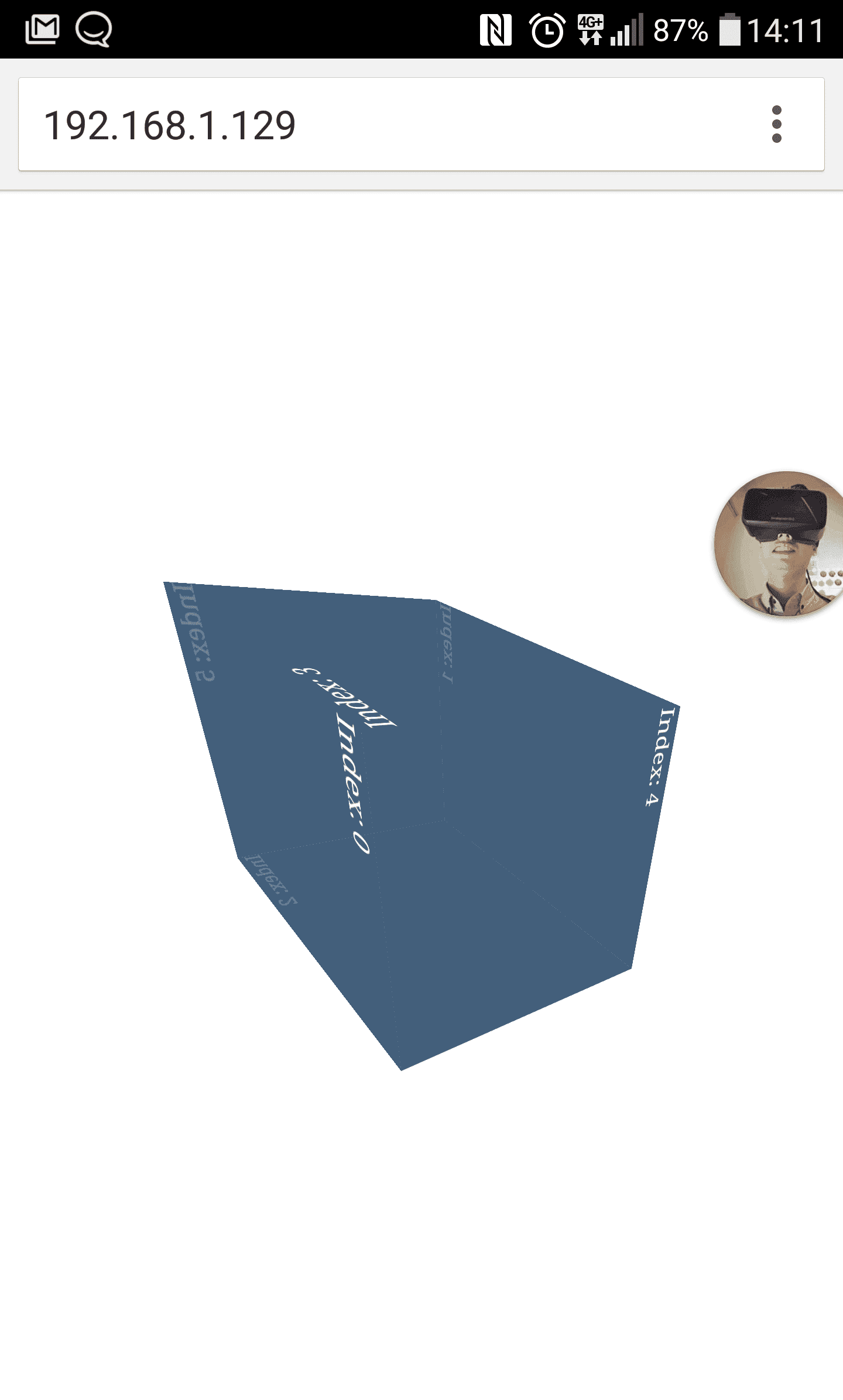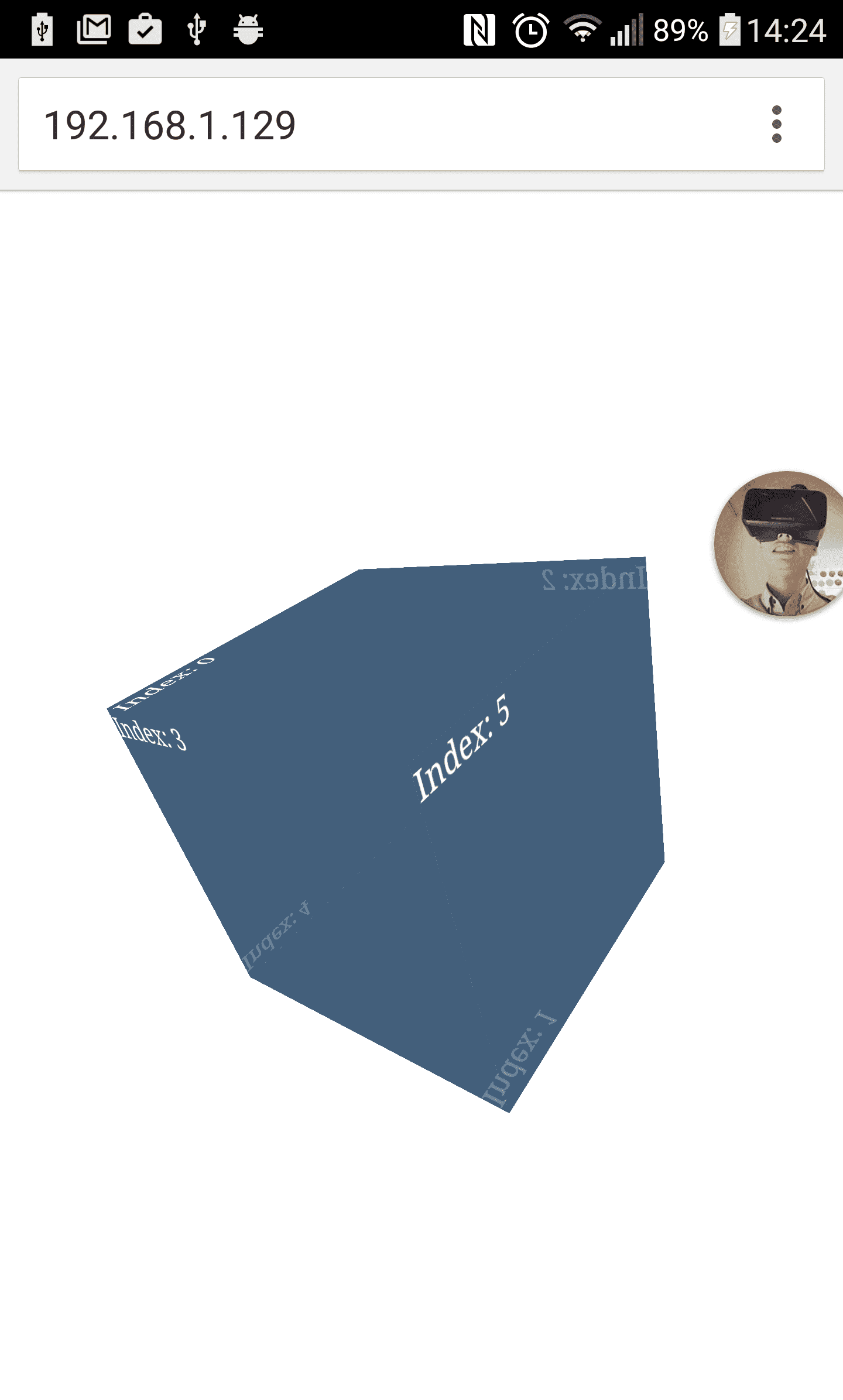Image source: http://heckifiknowcomics.com/post/134582065049

http://stackoverflow.com/questions/29716215/using-device-orientation-with-3d-transforms

## matrix3d

1. gamma，對於 Y 軸旋轉的角度變化
2. beta，對於 Z 軸旋轉的角度變化
3. alpha，對於 X 軸旋轉的角度變化

1. 開啟 3D MODE
2. X 的 ROTATE 輸入 170
3. X 的 ROTATE 輸入 180
4. X 的 ROTATE 輸入 190
5. X 的 ROTATE 輸入 0
6. Y 的 ROTATE 輸入 170
7. Y 的 ROTATE 輸入 180
8. Y 的 ROTATE 輸入 0
9. Z 的 ROTATE 輸入 170
10. Z 的 ROTATE 輸入 180

https://en.wikipedia.org/wiki/Rotation_matrix

http://www.w3.org/TR/css-transforms-1/#mathematical-description

A 3D rotation with the vector $[x, y, z]$ and the parameter $\alpha$ is equivalent to the matrix:

$$\begin{pmatrix} 1 - 2 * (y^2+z^2) * sq & 2 * (x * y * sq - z * sc) & 2 * (x * z * sq + y * sc) & 0\\ 2 * (x * y * sq + z * sc) & 1 - 2 * (x^2+z^2) * sq & 2 * (y * z * sq + x * sc) & 0\\ 2 * (x * z * sq - y * sc) & 2 * (y * z * sq + x * sc) & 1 - 2 * (x^2+y^2) * sq & 0\\ 0 & 0 & 0 & 1 \end{pmatrix}$$

$sc = sin(\alpha / 2) * cos(\alpha / 2)\\ sq = sin^2(\alpha / 2)$https://en.wikipedia.org/wiki/Euler_anglesBy Lionel Brits (Hand drawn in Inkscape by me) [GFDL (http://www.gnu.org/copyleft/fdl.html) or CC BY 3.0 (http://creativecommons.org/licenses/by/3.0)], via Wikimedia Commons

## rotate3dhttp://www.w3.org/Talks/2012/0416-CSS-WWW2012/Demos/transforms/demo-rotate3d.html

Watch out! vertical Y axis in 3d is inverted.

$$\begin{pmatrix} cos\theta + v_x^2 * (1 - cos\theta) & v_x * v_y * (1 - cos\theta) - v_z * sin\theta & v_x * v_z * (1 - cos\theta) + v_y * sin\theta\\ v_y * v_x * (1 - cos\theta) + v_z * sin\theta & cos\theta + v_y^2 * (1 - cos\theta) & v_y * v_z * (1 - cos\theta) - v_x * sin\theta\\ v_z * v_x * (1 - cos\theta) - v_y * sin\theta & v_z * v_x * (1 - cos\theta) + v_x * sin\theta & cos\theta + v_z^2 * (1 - cos\theta) \end{pmatrix}$$

1. $\alpha = [0, 360]$
2. $\beta = [-180, 180]$
3. $\gamma = [-90, 90]$By Euler2.gif: Juansempere derivative work: Xavax (This file was derived from Euler2.gif:) [CC BY-SA 3.0 (http://creativecommons.org/licenses/by-sa/3.0) or GFDL (http://www.gnu.org/copyleft/fdl.html)], via Wikimedia Commons

## 程式碼

// https://en.wikipedia.org/wiki/Rotation_matrix
// 向量座標軸轉換
function rotateMatrix(axis, angle) {
var sc = Math.cos(angle * Math.PI / 180.0),
sq = Math.sin(angle * Math.PI / 180.0),
x = axis,
y = axis,
z = axis;

var rm = [
[0,0,0],
[0,0,0],
[0,0,0],
];

rm = sc + x*x * (1-sc);
rm = z*sq + y*x * (1-sc);
rm = -y*sq + z*x * (1-sc);
rm = -z*sq + x*y * (1-sc);
rm = sc + y*y * (1-sc);
rm = x*sq + z*y * (1-sc);
rm = y*sq + x*z * (1-sc);
rm = -x*sq + y*z * (1-sc);
rm = sc + z*z * (1-sc);

return [
rm * vector + rm * vector + rm * vector,
rm * vector + rm * vector + rm * vector,
rm * vector + rm * vector + rm * vector
];
}

// 預設座標軸向量
var default_vector = {
x: [1, 0, 0],
y: [0, 1, 0],
z: [0, 0, 1],
};

window.ondeviceorientation = function(event) {
var alpha = Math.floor(event.alpha),
beta = Math.floor(event.beta),
gamma = Math.floor(event.gamma) * -1;

// 轉換座標軸向量
var axis = { x:0, y: 0, z: 0 };
// 用上面那個 GIF 圖片的順序來做轉換
axis.y = default_vecotr.y;
axis.x = rotateMatrix(default_vector.x, axis.y, gamma);
axis.z = rotateMatrix(rotateMatrix(default_vector.z, axis.y, gamma), axis.x, beta);

// 最後使用 rotate3d 寫入 transform
// 要注意的是，三軸順序是 Z -> X -> Y
// 等於將上面那張 GIF 圖片順序倒過來放
document.getElementById('#box').style.transform = 'rotate3d(' + axis.z + ', ' + axis.z + ', ' + axis.z + ', ' + alpha + 'deg) ' + 'rotate3d(' + axis.x + ', ' + axis.x + ', ' + axis.x + ', ' + beta + 'deg) ' + 'rotate3d(' + axis.y + ', ' + axis.y + ', ' + axis.y + ', ' + gamma + 'deg)';
};